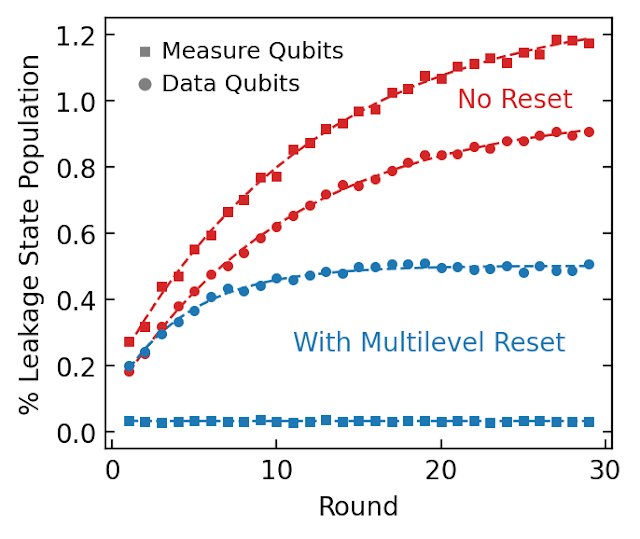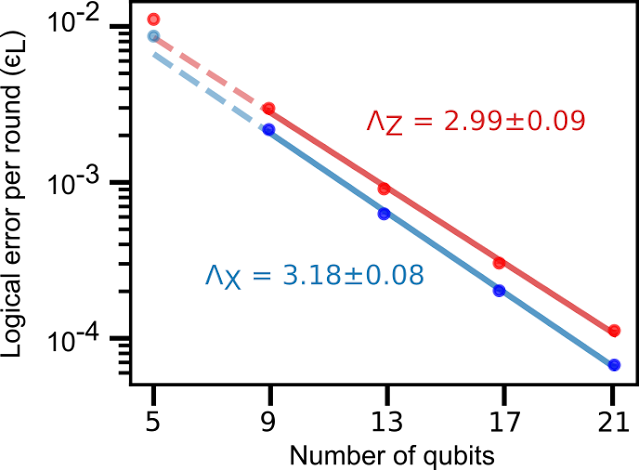# Demonstrating the Fundamentals of Quantum Error Correction

Posted by Jimmy Chen, Quantum Research Scientist and Matt McEwen, Student Researcher, Google Quantum AI

The Google Quantum AI team has been building quantum processors made of superconducting quantum bits (qubits) that have achieved the first beyond-classical computation, as well as the largest quantum chemical simulations to date. However, current generation quantum processors still have high operational error rates — in the range of 10-3 per operation, compared to the 10-12 believed to be necessary for a variety of useful algorithms. Bridging this tremendous gap in error rates will require more than just making better qubits — quantum computers of the future will have to use quantum error correction (QEC).

The core idea of QEC is to make a logical qubit by distributing its quantum state across many physical data qubits. When a physical error occurs, one can detect it by repeatedly checking certain properties of the qubits, allowing it to be corrected, preventing any error from occurring on the logical qubit state. While logical errors may still occur if a series of physical qubits experience an error together, this error rate should exponentially decrease with the addition of more physical qubits (more physical qubits need to be involved to cause a logical error). This exponential scaling behavior relies on physical qubit errors being sufficiently rare and independent. In particular, it’s important to suppress correlated errors, where one physical error simultaneously affects many qubits at once or persists over many cycles of error correction. Such correlated errors produce more complex patterns of error detections that are more difficult to correct and more easily cause logical errors.

Our team has recently implemented the ideas of QEC in our Sycamore architecture using quantum repetition codes. These codes consist of one-dimensional chains of qubits that alternate between data qubits, which encode the logical qubit, and measure qubits, which we use to detect errors in the logical state. While these repetition codes can only correct for one kind of quantum error at a time1, they contain all of the same ingredients as more sophisticated error correction codes and require fewer physical qubits per logical qubit, allowing us to better explore how logical errors decrease as logical qubit size grows.

In “Removing leakage-induced correlated errors in superconducting quantum error correction”, published in Nature Communications, we use these repetition codes to demonstrate a new technique for reducing the amount of correlated errors in our physical qubits. Then, in “Exponential suppression of bit or phase flip errors with repetitive error correction”, published in Nature, we show that the logical errors of these repetition codes are exponentially suppressed as we add more and more physical qubits, consistent with expectations from QEC theory.Layout of the repetition code (21 qubits, 1D chain) and distance-2 surface code (7 qubits) on the Sycamore device.

Leaky Qubits
The goal of the repetition code is to detect errors on the data qubits without measuring their states directly. It does so by entangling each pair of data qubits with their shared measure qubit in a way that tells us whether those data qubit states are the same or different (i.e., their parity) without telling us the states themselves. We repeat this process over and over in rounds that last only one microsecond. When the measured parities change between rounds, we’ve detected an error.

However, one key challenge stems from how we make qubits out of superconducting circuits. While a qubit needs only two energy states, which are usually labeled |0 and |1, our devices feature a ladder of energy states, |0, |1, |2, |3, and so on. We use the two lowest energy states to encode our qubit with information to be used for computation (we call these the computational states). We use the higher energy states (|2, |3 and higher) to help achieve high-fidelity entangling operations, but these entangling operations can sometimes allow the qubit to “leak” into these higher states, earning them the name leakage states.

Population in the leakage states builds up as operations are applied, which increases the error of subsequent operations and even causes other nearby qubits to leak as well — resulting in a particularly challenging source of correlated error. In our early 2015 experiments on error correction, we observed that as more rounds of error correction were applied, performance declined as leakage began to build.

Mitigating the impact of leakage required us to develop a new kind of qubit operation that could “empty out” leakage states, called multi-level reset. We manipulate the qubit to rapidly pump energy out into the structures used for readout, where it will quickly move off the chip, leaving the qubit cooled to the |0 state, even if it started in |2 or |3. Applying this operation to the data qubits would destroy the logical state we’re trying to protect, but we can apply it to the measure qubits without disturbing the data qubits. Resetting the measure qubits at the end of every round dynamically stabilizes the device so leakage doesn’t continue to grow and spread, allowing our devices to behave more like ideal qubits.Applying the multi-level reset gate to the measure qubits almost totally removes leakage, while also reducing the growth of leakage on the data qubits.

Exponential Suppression
Having mitigated leakage as a significant source of correlated error, we next set out to test whether the repetition codes give us the predicted exponential reduction in error when increasing the number of qubits. Every time we run our repetition code, it produces a collection of error detections. Because the detections are linked to pairs of qubits rather than individual qubits, we have to look at all of the detections to try to piece together where the errors have occurred, a procedure known as decoding. Once we’ve decoded the errors, we then know which corrections we need to apply to the data qubits. However, decoding can fail if there are too many error detections for the number of data qubits used, resulting in a logical error.

To test our repetition codes, we run codes with sizes ranging from 5 to 21 qubits while also varying the number of error correction rounds. We also run two different types of repetition codes — either a phase-flip code or bit-flip code — that are sensitive to different kinds of quantum errors. By finding the logical error probability as a function of the number of rounds, we can fit a logical error rate for each code size and code type. In our data, we see that the logical error rate does in fact get suppressed exponentially as the code size is increased.Probability of getting a logical error after decoding versus number of rounds run, shown for various sizes of phase-flip repetition code.

We can quantify the error suppression with the error scaling parameter Lambda (Λ), where a Lambda value of 2 means that we halve the logical error rate every time we add four data qubits to the repetition code. In our experiments, we find Lambda values of 3.18 for the phase-flip code and 2.99 for the bit-flip code. We can compare these experimental values to a numerical simulation of the expected Lambda based on a simple error model with no correlated errors, which predicts values of 3.34 and 3.78 for the bit- and phase-flip codes respectively.Logical error rate per round versus number of qubits for the phase-flip (X) and bit-flip (Z) repetition codes. The line shows an exponential decay fit, and Λ is the scale factor for the exponential decay.

This is the first time Lambda has been measured in any platform while performing multiple rounds of error detection. We’re especially excited about how close the experimental and simulated Lambda values are, because it means that our system can be described with a fairly simple error model without many unexpected errors occurring. Nevertheless, the agreement is not perfect, indicating that there’s more research to be done in understanding the non-idealities of our QEC architecture, including additional sources of correlated errors.

What’s Next
This work demonstrates two important prerequisites for QEC: first, the Sycamore device can run many rounds of error correction without building up errors over time thanks to our new reset protocol, and second, we were able to validate QEC theory and error models by showing exponential suppression of error in a repetition code. These experiments were the largest stress test of a QEC system yet, using 1000 entangling gates and 500 qubit measurements in our largest test. We’re looking forward to taking what we learned from these experiments and applying it to our target QEC architecture, the 2D surface code, which will require even more qubits with even better performance.

1A true quantum error correcting code would require a two dimensional array of qubits in order to correct for all of the errors that could occur.Read More